## Is there something out there?

### Introduction: you said “space”?

high-dimensional sensory input vector$\qquad \overset{\text{Brain}}{\rightsquigarrow} \qquad \underbrace{\textit{space, attributes, ...}}_{\text{easier to visualize}}$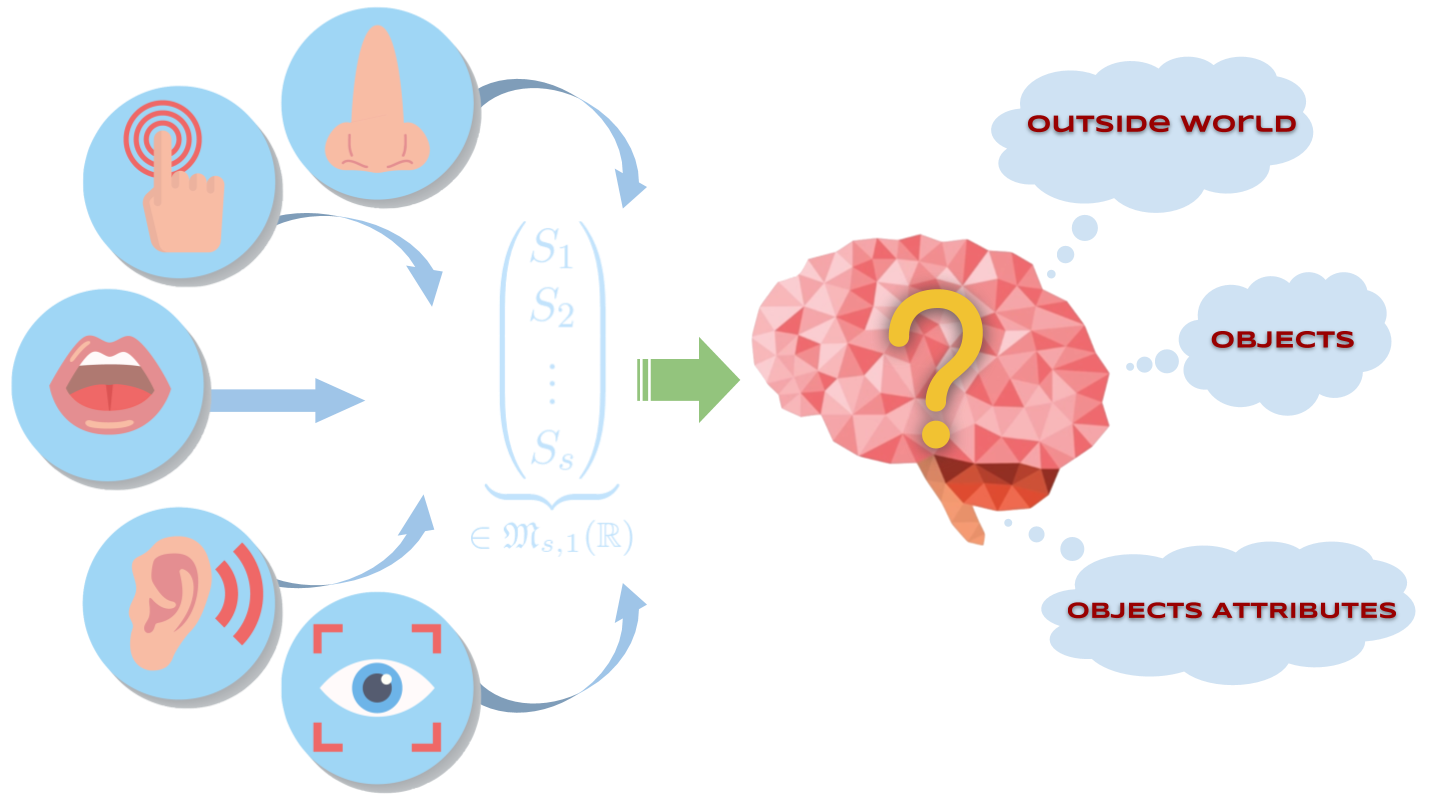All the brain can do:
1. issue motor commands

2. observe the resulting environmental changes

then collect sensory inputs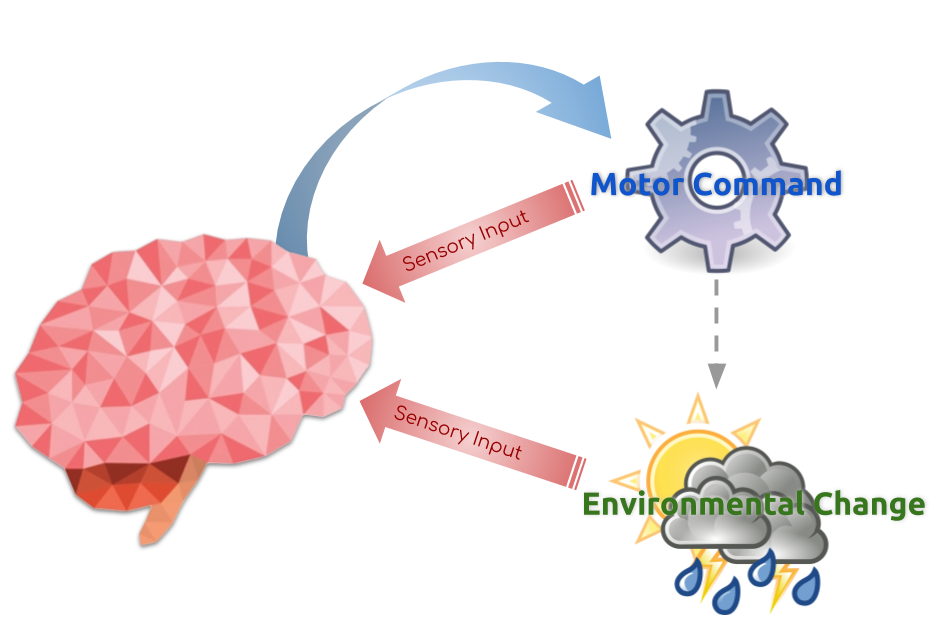## I. - Exteroception & Compensation

### I.A Exteroception vs. Proprioception

Sensory input Definition
Proprioceptive independent of the environment
Exteroceptive dependent of the environment

### Example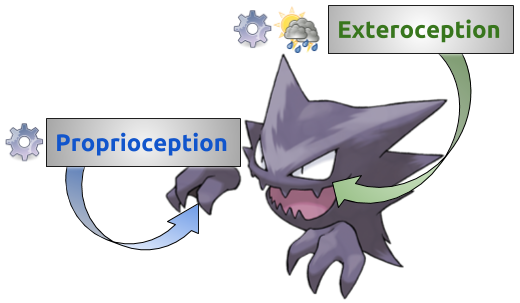### I.B - Compensated movements

Compensated movements:
Variations of the motor command and the environment that compensate one another.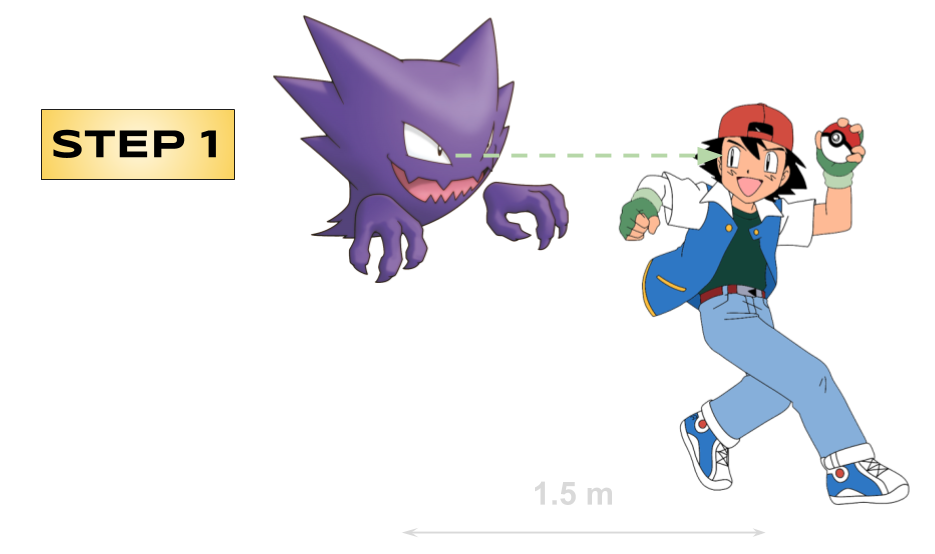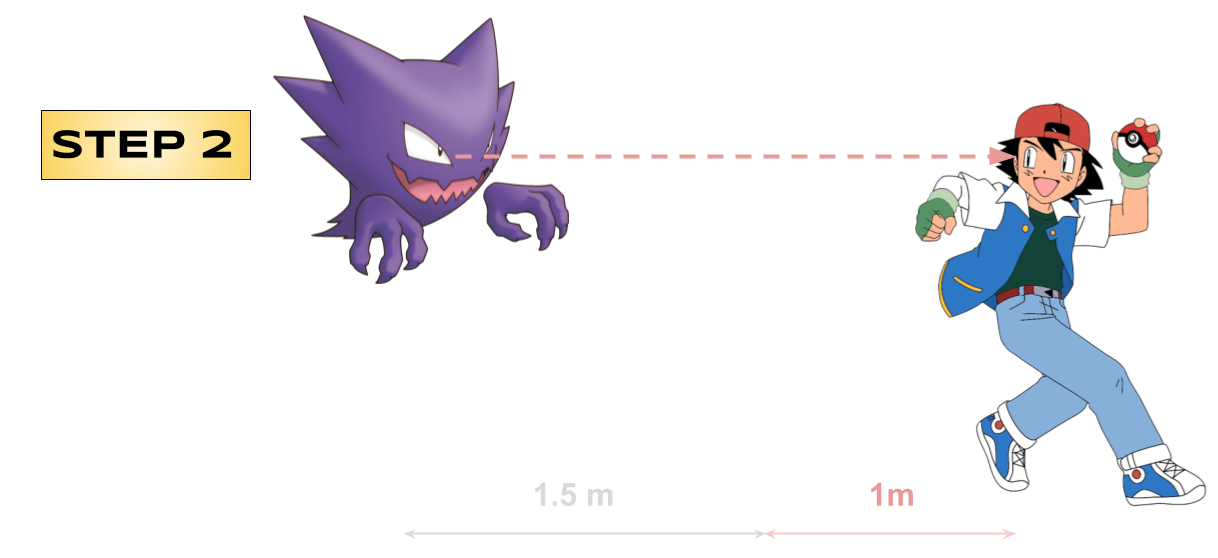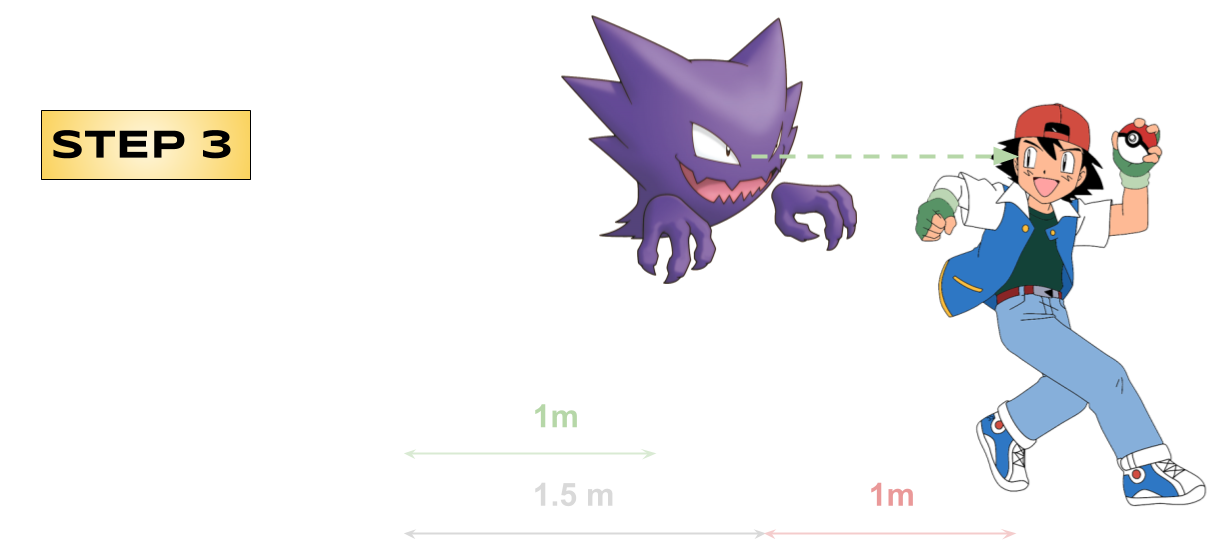Relative distance between them is the same at steps 1 & 3

### Organism 1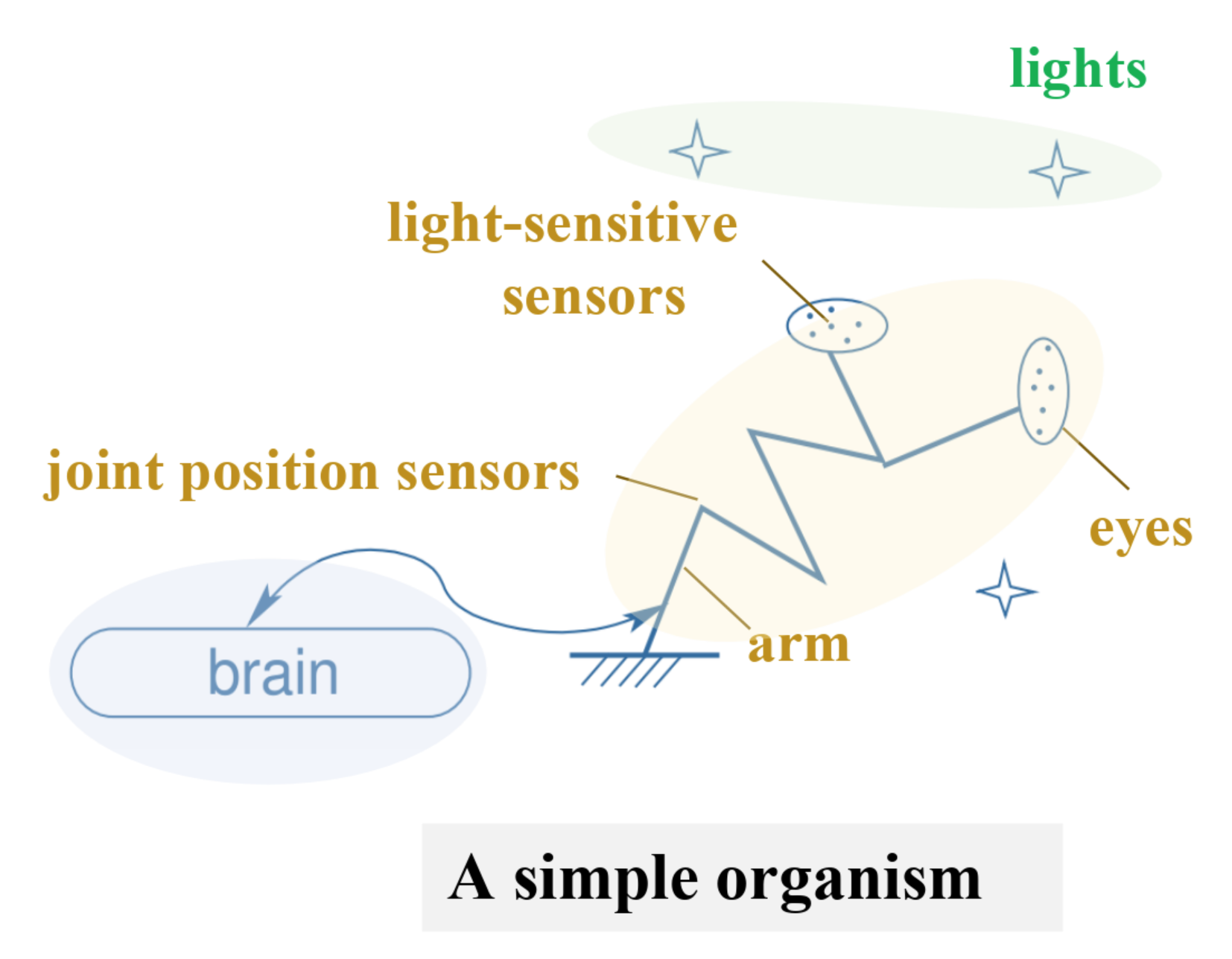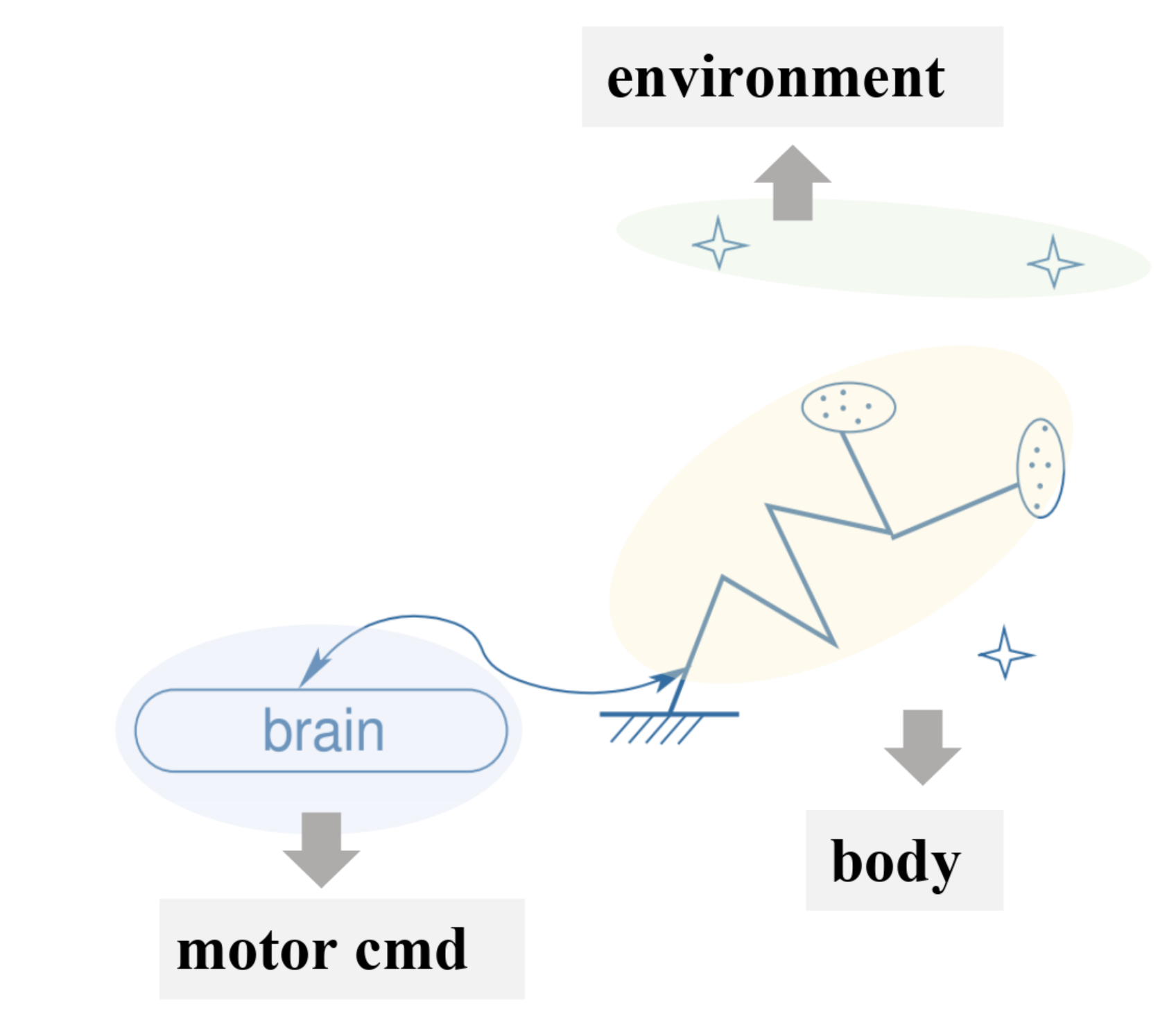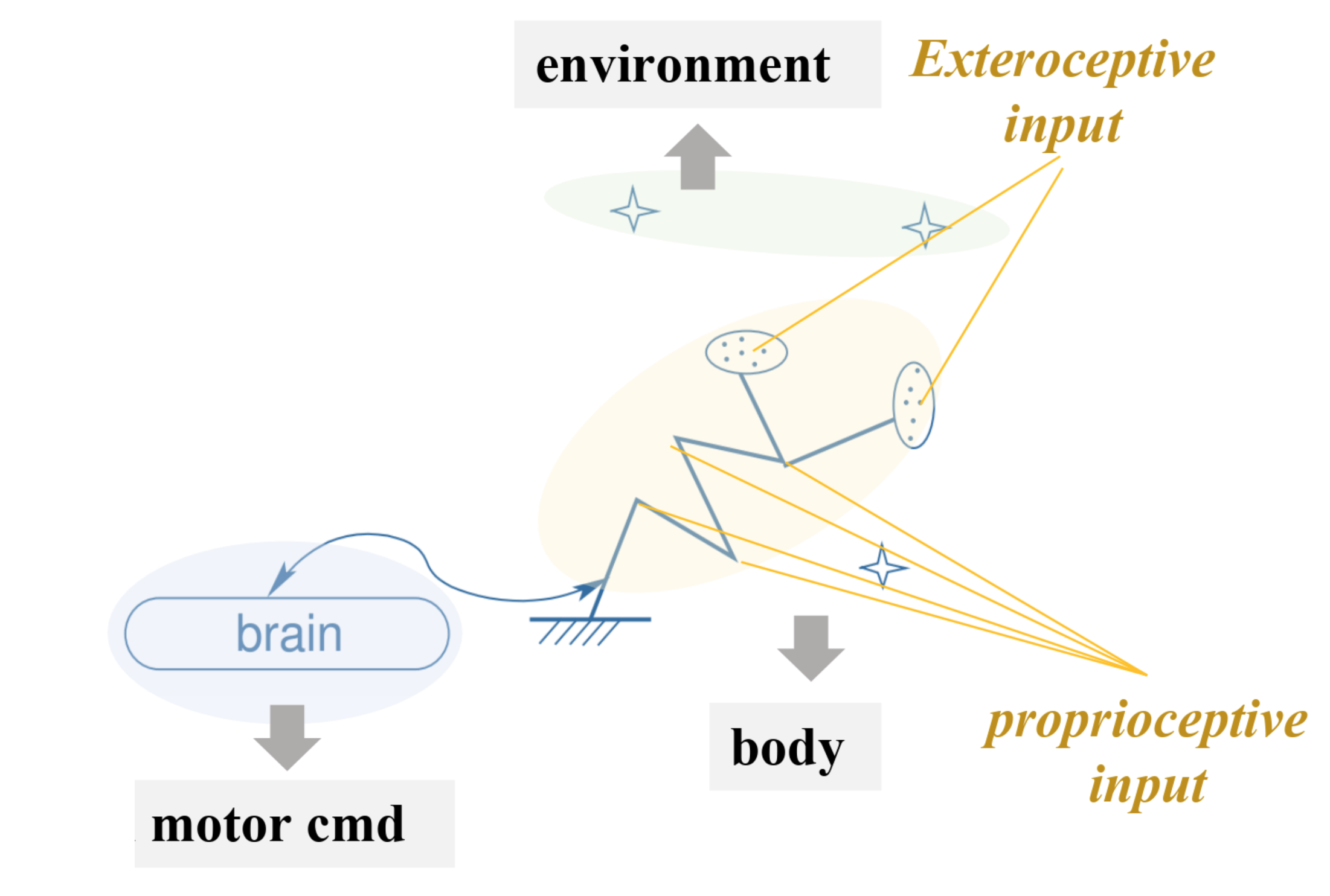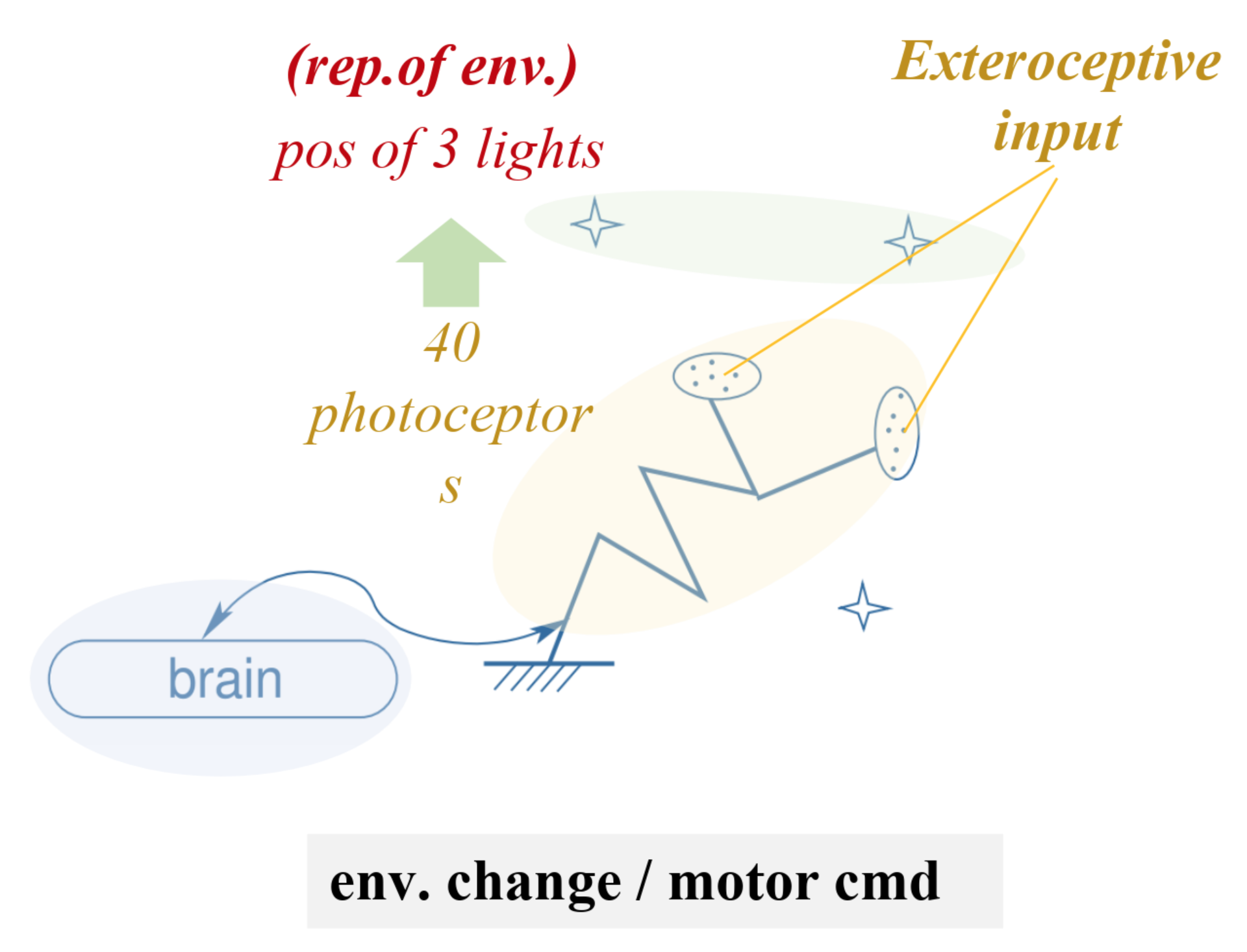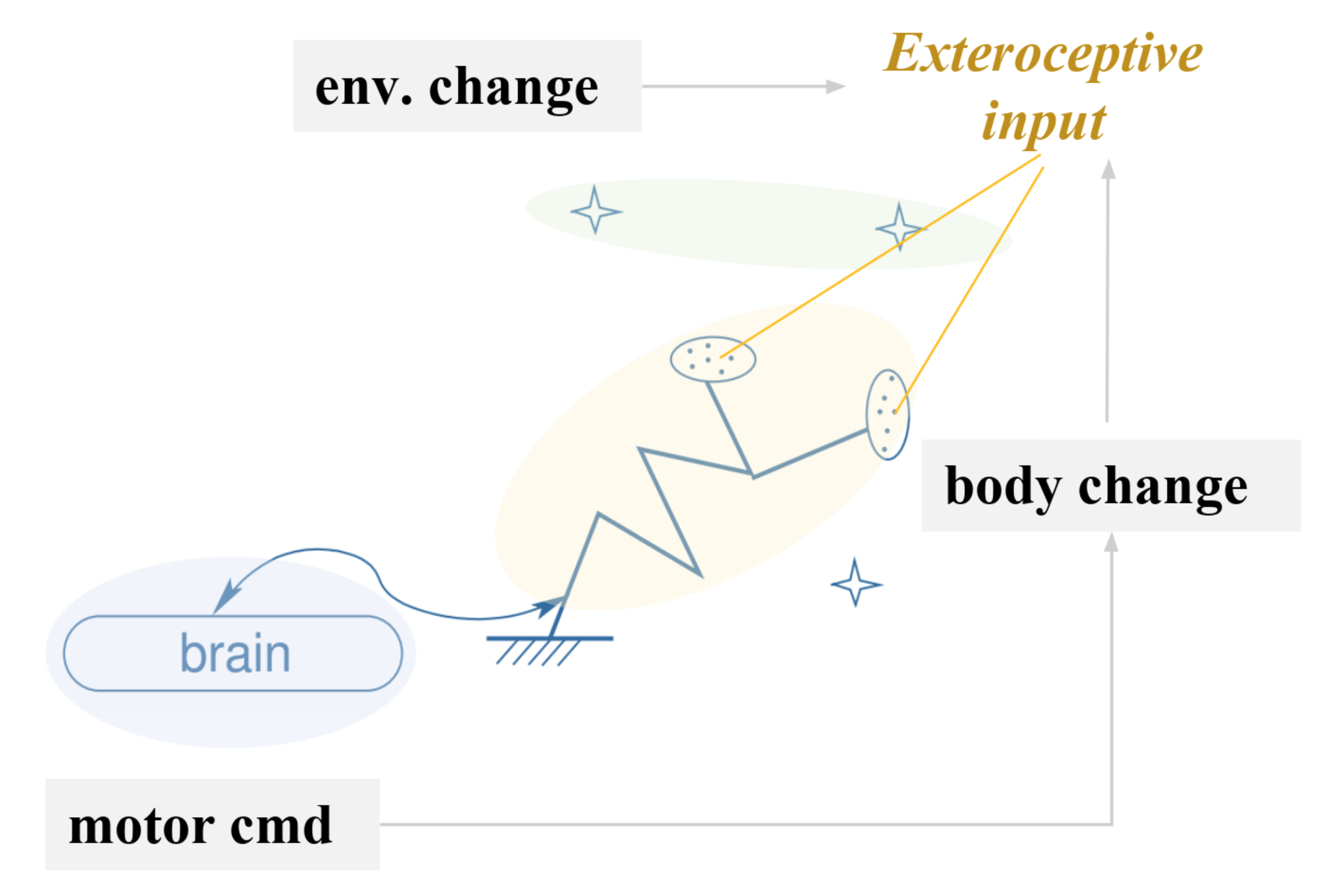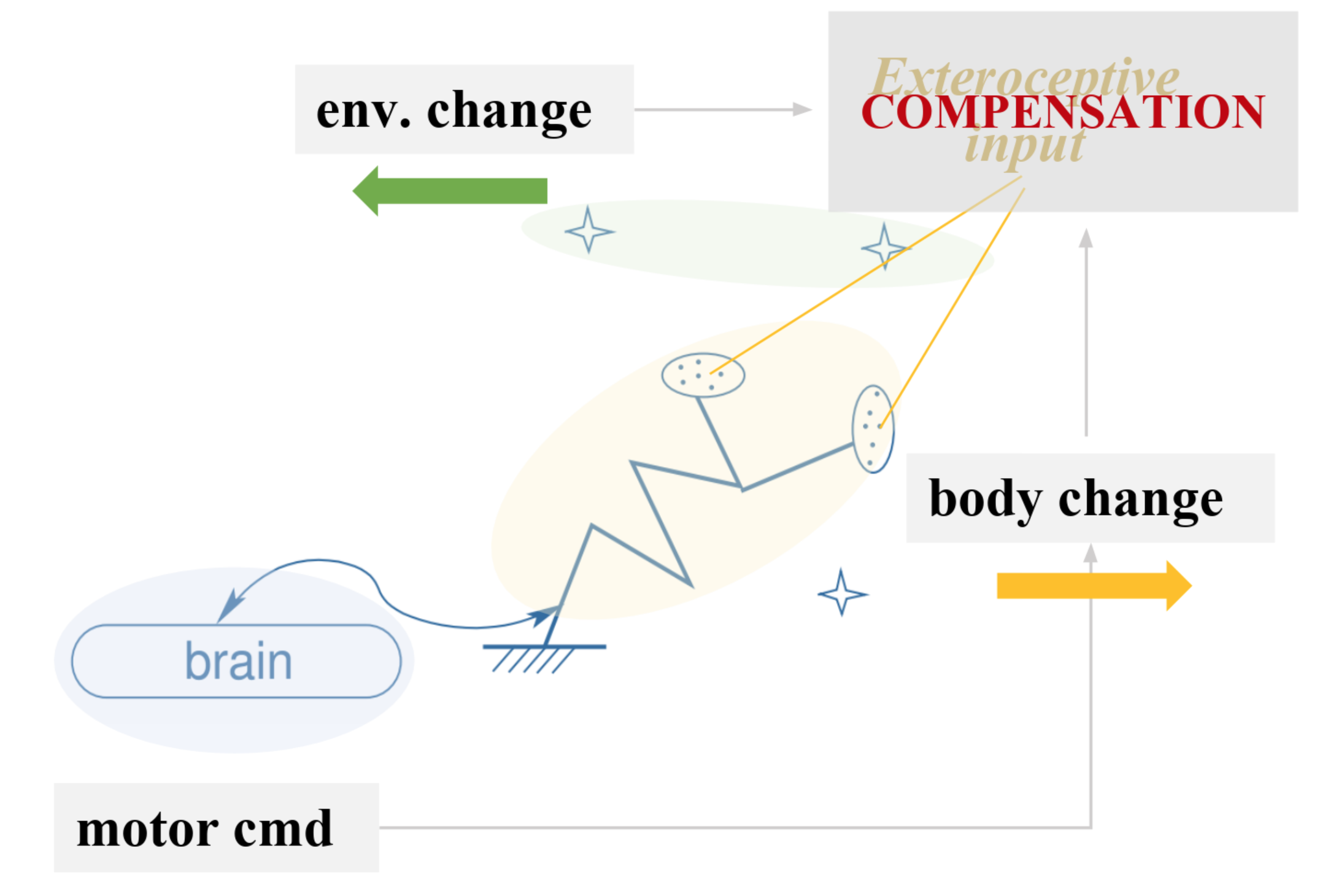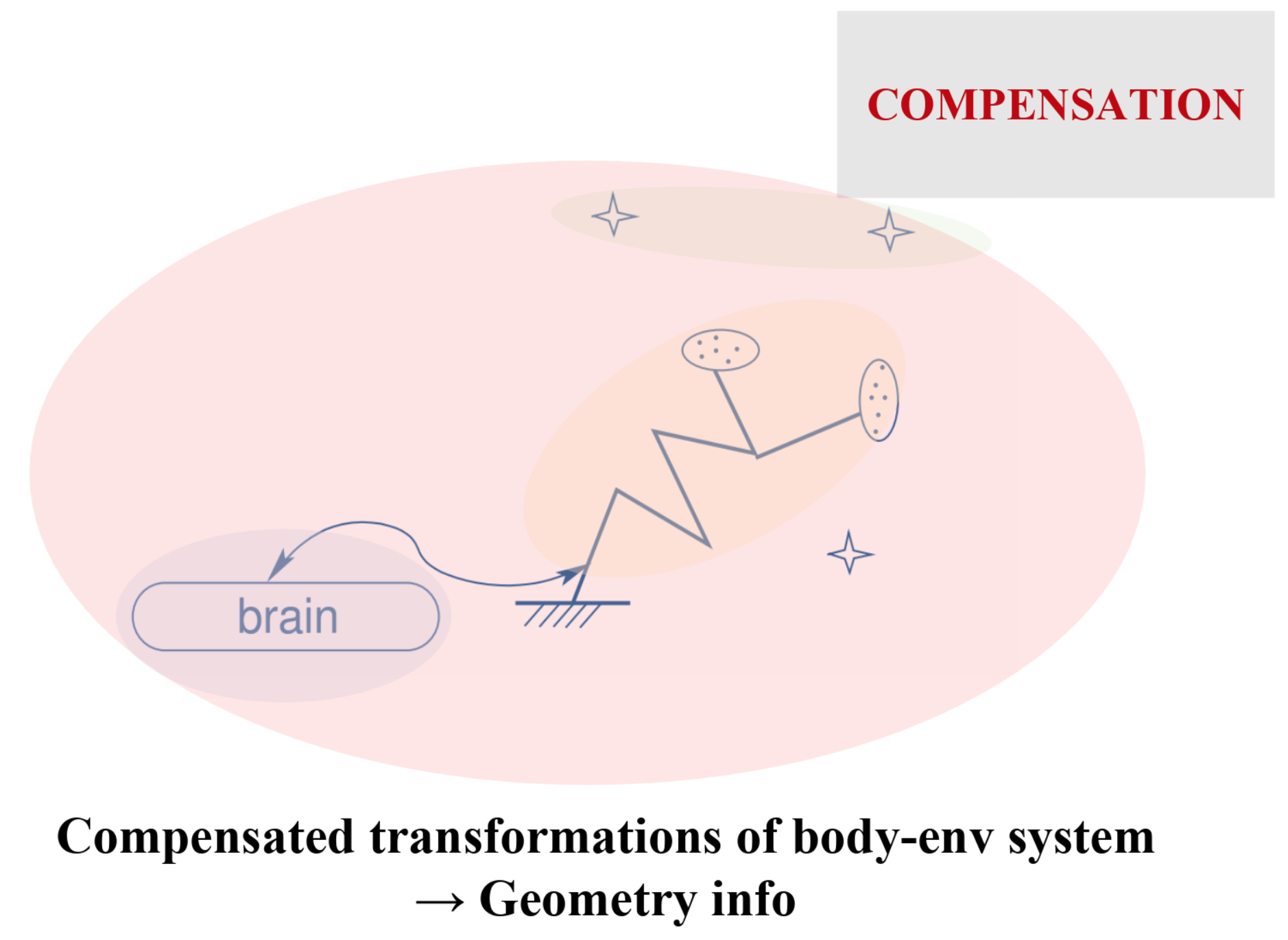Compensable movements: exactly what stems from the notion of the physical space in the sensory inputs

So the true goal: computing the dimension of the rigid group of compensated movements.

### II. Mathematical formulation

\begin{align*} \mathcal{E} &≝ \lbrace E ∈ \text{environmental states}\rbrace\\ \mathcal{M} &≝ \lbrace M ∈ \text{motor commands}\rbrace\\ \mathcal{S} &≝ \lbrace S ∈ \text{sensory inputs}\rbrace \end{align*}

are manifolds of dimension $e, m$ and $s$ respectively such that:

$$\mathcal{S} = ψ(\mathcal{M} × \mathcal{E})$$

NB: We are only considering exteroceptive inputs, i.e. points $S^e ∈ \mathcal{S}$ s.t.:

$$∃ \mathcal{M}' ⊆ \mathcal{M}; \; ψ^{-1}(S^e) = \mathcal{M}' × \mathcal{E}$$

Pushforward of $(M_0, E_0)$ by $ψ$

⟹ Tangent space at $S_0 ≝ ψ(M_0, E_0)$:

$$\lbrace dS \rbrace = \lbrace dS \rbrace_{dE=0} + \lbrace dS \rbrace_{dM=0}$$

Moreover:

• $\lbrace dS \rbrace_{dE=0}$ is the tangent space of $ψ(E_0, \mathcal{M})$ at $S_0$
• $\lbrace dS \rbrace_{dM=0}$ is the tangent space of $ψ(\mathcal{E}, M_0)$ at $S_0$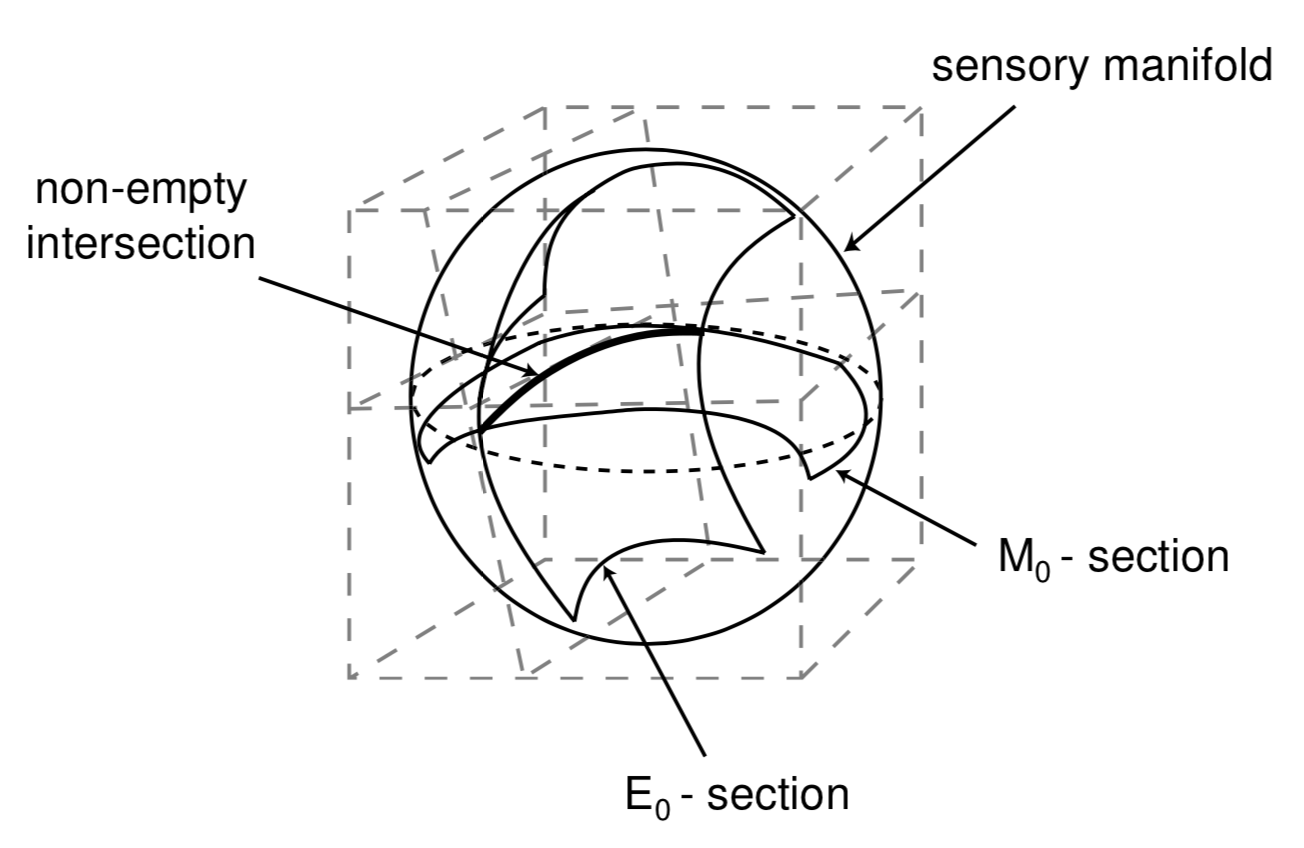$$\mathcal{C}(M_0, E_0) ≝ ψ(\mathcal{E}, M_0) ∩ ψ(\mathcal{E}, M_0)$$

Along $\mathcal{C}(M_0, E_0)$: exteroceptive changes obtained by adding

• either $dE$
• or $dM$.

Compensated (infinitesimal) movements:
when infinitesimal changes along $\lbrace dS \rbrace_{dE=0}$ and $\lbrace dS \rbrace_{dM=0}$ compensate one another

Dimension of the space of compensated movements:
$$d ≝ \dim \underbrace{\lbrace dS_{dM=0} \mid ∃ dS_{dE=0}; dS_{dM=0} + dS_{dE=0} = 0 \rbrace}_{= \; \lbrace dS \rbrace_{dE=0} ∩ \lbrace dS \rbrace_{dM=0}} = \dim \mathcal{C}(M_0, E_0)$$

So by Grassmann formula:

\begin{align*} d \quad &≝ \quad \dim \lbrace dS \rbrace_{dE=0} ∩ \lbrace dS \rbrace_{dM=0}\\ \quad &= \quad \dim \lbrace dS \rbrace_{dE=0} + \dim \lbrace dS \rbrace_{dM=0} \\ \quad & \qquad - \dim \Big( \underbrace{\lbrace dS \rbrace_{dE=0} +\lbrace dS \rbrace_{dM=0}}_{= \lbrace dS \rbrace} \Big)\\ \\ \quad &= \quad \dim \lbrace dS \rbrace_{dE=0} + \dim \lbrace dS \rbrace_{dM=0} - \dim (\lbrace dS \rbrace) \end{align*}

## Algorithm

Get rid of proprioceptive inputs  # (these don't change when no motor command is issued and the environment changes)

for "source" in [motor commands, environment, both]:
Estimate dim(space of sensory inputs resulting from "source" variations)

dim(compensated movements) =   dim(inputs resulting from motor commands variations)
+ dim(inputs resulting from environment variations)
- dim(inputs resulting from both variations)


### Principal Component Analysis

Goal: Find orthogonal axes onto which the variance of the data points under projection is maximal, i.e. find the best possible “angles” from which the data points are the most spread out.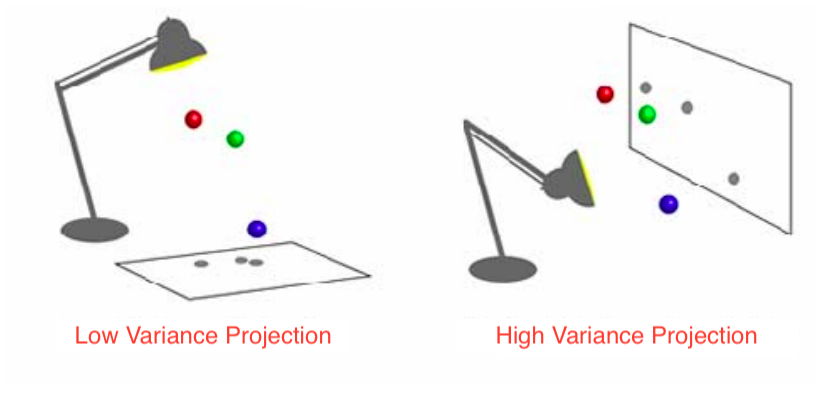### Implementation

Neurorobotics_Project
│   index.md
│
└───sensorimotor_dependencies
│   │   __init__.py
│   │   utils.py
│   │   organisms.py
│
└───docs
│   ...


where

- utils.py ⟹ utility functions, among which dimension reduction algorithms
- organisms.py ⟹ Organism1(), Organism2(), Organism3()


### Object-Oriented Programming

class Organism1:
def __init__(self, seed=1, retina_size=1., M_size=M_size, E_size=E_size,
nb_joints=nb_joints, nb_eyes=nb_eyes, nb_lights=nb_lights,
extero=extero, proprio=proprio,
nb_generating_motor_commands=nb_generating_motor_commands,
nb_generating_env_positions=nb_generating_env_positions,
neighborhood_size=neighborhood_size, sigma=σ):

self.random = np.random.RandomState(seed)
# [...]
self.random_state = self.random.get_state()

def get_sensory_inputs(self, M, E, QPaL=None):
# [...]

def get_proprioception(self):
# [...]

def get_variations(self):
self.env_variations = ...
self.mot_variations = ...
self.env_mot_variations = ...

def get_dimensions(self, dim_red='PCA'):
self.get_proprioception()
self.get_variations()
# Now the number of degrees of freedom!
self.dim_env = dim_reduction_dict[dim_red](self.env_variations)
self.dim_extero = ...
self.dim_env_extero = ...
self.dim_rigid_group = ...

return self.dim_rigid_group, self.dim_extero, self.dim_env, self.dim_env_extero


### Other organisms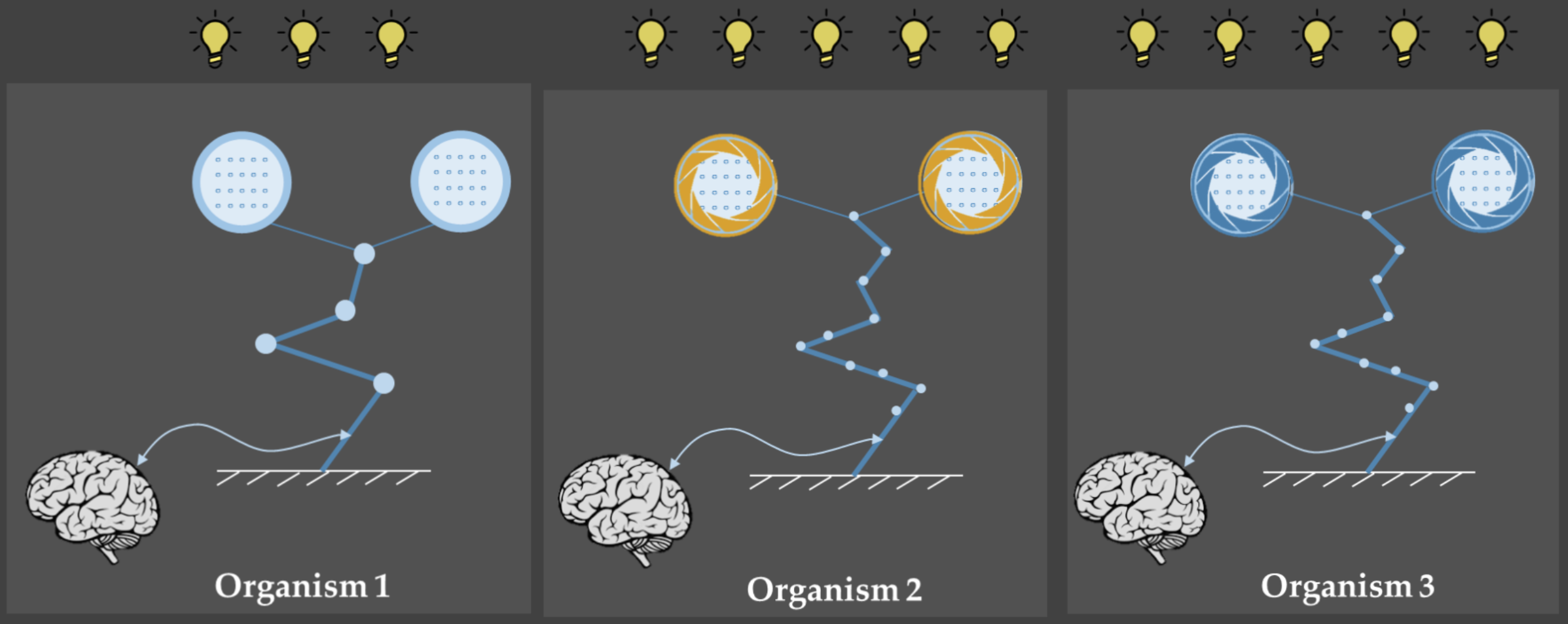>>>  O = organisms.Organism1(); O.get_dimensions()
(4, 10, 5, 11)

>>> print(str(O))

Characteristics Value
Dimension of motor commands 40
Dimension of environmental control vector 40
Dimension of proprioceptive inputs 16
Dimension of exteroceptive inputs 40
Number of eyes 2
Number of joints 4
Diaphragms None
Number of lights 3
Light luminance Fixed
Dimension for body § 10
Dimension for environment (e) 5
Dimension for both (b) 11
Dimension of group of compensated movements 4

## Some results

Varying retina_size (called var) values for different random seeds.